# excel统计个数方法汇总

[日期：2011-10-25]   来源：IT部落窝  作者：IT部落窝   阅读：52199[字体： ]

excel统计个数在excel中的应用是极其常见的。当然excel统计个数的条件也是多种多样，本文就典型的excel统计个数，给出一些相关案例，共同学习。

首先来看看，excel统计个数不使用公式，使用状态栏提示快速统计字符个数。当打开excel表格后，选中要统计的数据区域，这时请观察状态栏，状态栏里是显示出统计数字的。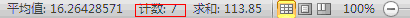提示：如果在excel工作表中选中的文本单元格，会提示计数，如果选中的是数字区域，则会出现求平均、计数、求和等等。

excel统计个数实例一：统计A列类列ABCD对应的数量大于0的个数。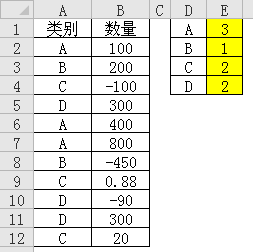该题excel统计个数的公式的解法：
一：=COUNTIFS(A:A,D1,B:B,">0")
二：=SUMPRODUCT((\$A\$2:\$A\$12=D1)*(\$B\$2:\$B\$12>0))
三：选中E1：E4单元格，输入公式数组公式：=MMULT(N(TRANSPOSE(A2:A12)=D1:D4),N(B2:B12>0))

excel统计个数实例二：统计A列不重复的公司个数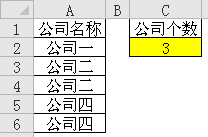该题excel统计个数的公式的解法：
一：数组公式：=SUM(1/COUNTIF(A2:A6,A2:A6))
二：普通公式：=SUMPRODUCT(1/COUNTIF(A2:A6,A2:A6))

excel统计个数实例三：统计时间段内正数个数

Excel统计条件：统计汪洋在开始和截止日期之间的正数个数。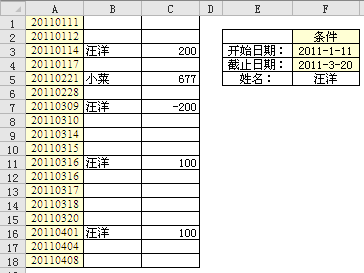该题excel统计个数的公式的解法：
一：=SUMPRODUCT((B1:B18=F5)*(C1:C18>0)*(A1:A18>=F3)*(A1:A18<=F4))
二：=COUNTIFS(B:B,F5,C:C,">0",A:A,">="&F3,A:A,"<="&F4)

excel统计个数实例四：上下行统计不重复数据

如下图所示：左边是数据，右边是模拟结果。查找上下行数据，并将不重复的数据统计出来。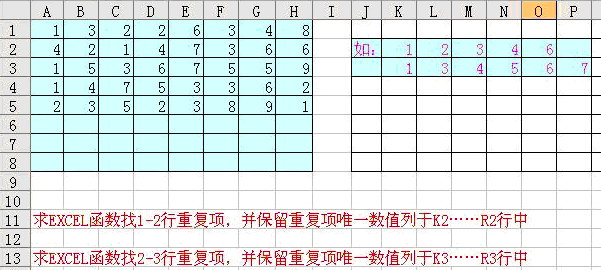数组公式：=TEXT(SMALL(IF(COUNTIF(\$A1:\$H2,ROW(\$1:\$9))>1,ROW(\$1:\$9),10),COLUMN(A1)),"[<10];")，右拉下拉复制即可统计出来。

excel统计个数确实很常用，除了上面用函数公式完成统计个数，另外还可以使用数据透视表来完成excel统计个数。分享到： QQ空间 新浪微博 腾讯微博 人人网